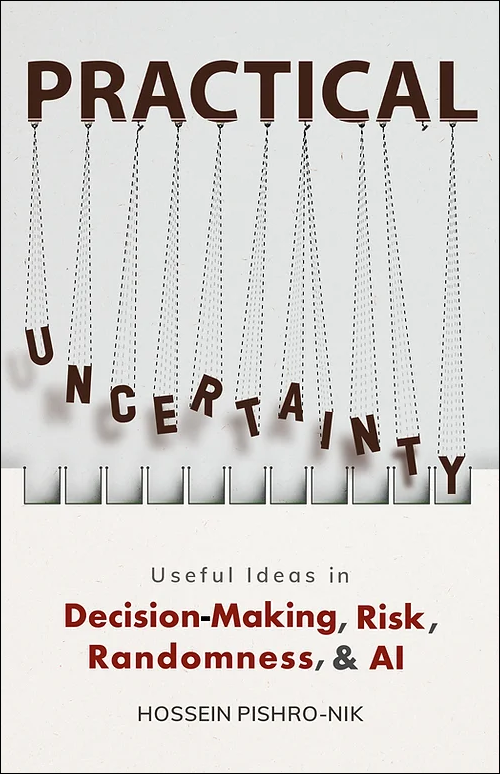## 4.2.2 Exponential Distribution

The exponential distribution is one of the widely used continuous distributions. It is often used to model the time elapsed between events. We will now mathematically define the exponential distribution, and derive its mean and expected value. Then we will develop the intuition for the distribution and discuss several interesting properties that it has.

A continuous random variable $X$ is said to have an exponential distribution with parameter $\lambda>0$, shown as $X \sim Exponential(\lambda)$, if its PDF is given by \begin{equation} \nonumber f_X(x) = \left\{ \begin{array}{l l} \lambda e^{-\lambda x} & \quad x > 0\\ 0 & \quad \textrm{otherwise} \end{array} \right. \end{equation}

Figure 4.5 shows the PDF of exponential distribution for several values of $\lambda$.

It is convenient to use the unit step function defined as \begin{equation} \nonumber u(x) = \left\{ \begin{array}{l l} 1 & \quad x \geq 0\\ 0 & \quad \textrm{otherwise} \end{array} \right. \end{equation} so we can write the PDF of an $Exponential(\lambda)$ random variable as $$f_X(x)= \lambda e^{-\lambda x} u(x).$$

Let us find its CDF, mean and variance. For $x > 0$, we have $$F_X(x) = \int_{0}^{x} \lambda e^{-\lambda t}dt=1-e^{-\lambda x}.$$ So we can express the CDF as $$F_X(x) = \big(1-e^{-\lambda x}\big)u(x).$$

Let $X \sim Exponential (\lambda)$. We can find its expected value as follows, using integration by parts:

 $EX$ $= \int_{0}^{\infty} x \lambda e^{- \lambda x}dx$ $= \frac{1}{\lambda} \int_{0}^{\infty} y e^{- y}dy$ $\textrm{choosing$y=\lambda x$}$ $= \frac{1}{\lambda} \bigg[-e^{-y}-ye^{-y} \bigg]_{0}^{\infty}$ $=\frac{1}{\lambda}.$

Now let's find Var$(X)$. We have

 $EX^2$ $= \int_{0}^{\infty} x^2 \lambda e^{- \lambda x}dx$ $= \frac{1}{\lambda^2} \int_{0}^{\infty} y^2 e^{- y}dy$ $= \frac{1}{\lambda^2} \bigg[-2e^{-y}-2ye^{-y}-y^2e^{-y} \bigg]_{0}^{\infty}$ $=\frac{2}{\lambda^2}.$

Thus, we obtain $$\textrm{Var} (X)=EX^2-(EX)^2=\frac{2}{\lambda^2}-\frac{1}{\lambda^2}=\frac{1}{\lambda^2}.$$

If $X \sim Exponential(\lambda)$, then $EX=\frac{1}{\lambda}$ and Var$(X)=\frac{1}{\lambda^2}$.

An interesting property of the exponential distribution is that it can be viewed as a continuous analogue of the geometric distribution. To see this, recall the random experiment behind the geometric distribution: you toss a coin (repeat a Bernoulli experiment) until you observe the first heads (success). Now, suppose that the coin tosses are $\Delta$ seconds apart and in each toss the probability of success is $p=\Delta \lambda$. Also suppose that $\Delta$ is very small, so the coin tosses are very close together in time and the probability of success in each trial is very low. Let $X$ be the time you observe the first success. We will show in the Solved Problems section that the distribution of $X$ converges to $Exponential(\lambda)$ as $\Delta$ approaches zero.

To get some intuition for this interpretation of the exponential distribution, suppose you are waiting for an event to happen. For example, you are at a store and are waiting for the next customer. In each millisecond, the probability that a new customer enters the store is very small. You can imagine that, in each millisecond, a coin (with a very small $P(H)$) is tossed, and if it lands heads a new customers enters. If you toss a coin every millisecond, the time until a new customer arrives approximately follows an exponential distribution.

The above interpretation of the exponential is useful in better understanding the properties of the exponential distribution. The most important of these properties is that the exponential distribution is memoryless. To see this, think of an exponential random variable in the sense of tossing a lot of coins until observing the first heads. If we toss the coin several times and do not observe a heads, from now on it is like we start all over again. In other words, the failed coin tosses do not impact the distribution of waiting time from now on. The reason for this is that the coin tosses are independent. We can state this formally as follows: $$P(X > x+a |X > a)=P(X > x).$$

If $X$ is exponential with parameter $\lambda>0$, then $X$ is a memoryless random variable, that is $$P(X > x+a \hspace{5pt} | \hspace{5pt} X > a)=P(X > x), \hspace{10pt} \textrm{ for }a,x \geq 0.$$

From the point of view of waiting time until arrival of a customer, the memoryless property means that it does not matter how long you have waited so far. If you have not observed a customer until time $a$, the distribution of waiting time (from time $a$) until the next customer is the same as when you started at time zero. Let us prove the memoryless property of the exponential distribution. \begin{align}%\label{} \nonumber P(X > x+a |X > a) &= \frac{P\big(X > x+a, X > a \big)}{P(X > a)}\\ \nonumber &= \frac{P(X > x+a)}{P(X > a)}\\ \nonumber &= \frac{1-F_X(x+a)}{1-F_X(a)}\\ \nonumber &= \frac{e^{-\lambda (x+a)}}{e^{-\lambda a}}\\ \nonumber &= e^{-\lambda x}\\ \nonumber &= P(X > x).\\ \end{align}

 The print version of the book is available on Amazon.Practical uncertainty: Userful Ideas in Decision-Making, Risk, Randomness, & AI